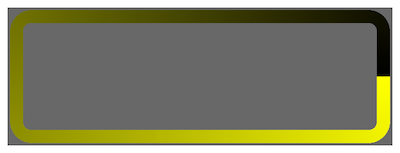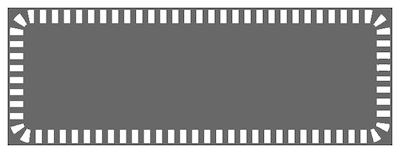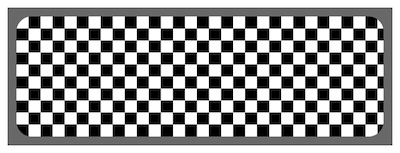# Controlling Shape Drawing with Shaders

Change a shape node’s appearance by supplying custom shader code.

### Overview

When you want to go beyond the effects provided by a shape node’s properties, you can take full control of its stroking or filling by using the `strokeShader` and `fillShader` properties, respectively. To do that, you supply custom OpenGL ES shader code embedded within a `SKShader` object. Custom shaders allow you to create custom effects, such as dashed lines and gradient strokes, and custom fills, such as checkerboards and random patterns.

### Customize a Shape Node’s Stroke

Shape nodes have two additional stroke-related properties that extend the properties defined by `SKShader`:

Symbol declaration Type Description
float u_path_length; Uniform The total length of the path, in points.
float v_path_distance; Varying The distance along the path, in points.

By dividing the distance along the path by the total length of the path, you get the normalized position (between 0 and 1) of each point along a shape node’s path and use it to construct the color of each pixel along the shape node’s stroke. The following code shows how you create a custom shader to do this:

``````let gradientShader = SKShader(source: "void main() {" +
"float normalisedPosition = v_path_distance / u_path_length;" +
"gl_FragColor = vec4(normalisedPosition, normalisedPosition, 0.0, 1.0);" +
"}")
let squareShapeNode = SKShapeNode(rectOf: CGSize(width: 610, height: 200),
cornerRadius: 25)
squareShapeNode.fillColor = .clear
squareShapeNode.lineWidth = 20
squareShapeNode.strokeShader = gradientShader
``````

The generated shape node looks like this:Alternatively, by casting both symbols to integers and using the modulo operator, you get the same shape node with a shader that generates a dashed line, as shown in the following code:

``````let dashedShader = SKShader(source: "void main() {" +
"int stripe = int(u_path_length) / 150;" +
"int h = int(v_path_distance) / stripe % 2;" +
"gl_FragColor = float4(h);" +
"}")
``````

The generated shape node looks like this:### Customize a Shape Node’s Fill

You create a custom fill for a shape node by writing shader code and embedding it within an `SKShader` object. Assigning the shader to the `fillShader` property overrides the appearance that would otherwise be defined by `fillColor` and `fillTexture`.

The following shader code demonstrates filling a shape node with a simple checkerboard texture. Inside the shader, the variables h and v would, on their own, form horizontal and vertical stripes. The exclusive or operator, ^, creates the checkerboard pattern from those stripes.

``````let checkerboardShader = SKShader(source: "void main() {" +
"int size = 20;" +
"int h = int(v_tex_coord.x * u_texture_size.x) / size % 2;" +
"int v = int(v_tex_coord.y * u_texture_size.y) / size % 2;" +
"gl_FragColor = float4(v ^ h, v ^ h, v ^ h, 1.0);" +
"}")

let size = CGSize(width: 610, height: 200)

checkerboardShader.uniforms = [
SKUniform(name: "u_texture_size",
vectorFloat2: vector_float2(Float(size.width), Float(size.height)))
]

let squareShapeNode = SKShapeNode(rectOf: size,
cornerRadius: 25)
squareShapeNode.fillShader = checkerboardShader
``````

The generated shape node looks like this:download this page as .md

download this page as .pdf

back to SpriteKit documentation